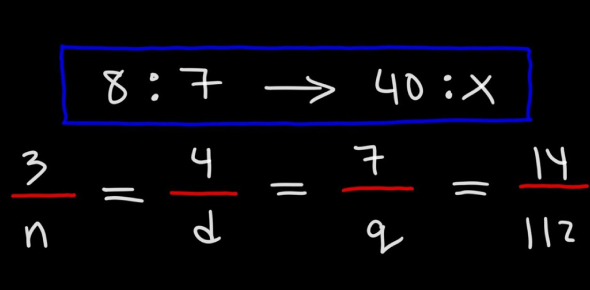# Ratio And Proportion MCQ Exam: Quiz!

10 Questions | Total Attempts: 38Settings.

• 1.
Rs.432 is divided amongst three workers A, B and C such that 8 times A’s share is equal to 12 times B’s share which is equal to 6 times C’s share. How much did A get?
• A.

Rs 192

• B.

Rs 133

• C.

Rs 144

• D.

Rs 128

• 2.
The monthly salaries of two persons are in the ratio of 4: 7. If each receives an increase of Rs.25 in the salary, the ratio is altered to 3: 5. Find their respective salaries.
• A.

120 & 210

• B.

80 & 140

• C.

180 & 300

• D.

200 & 350

• 3.
A sum of money is to be distributed among A, B, C, D in the proportion of 5 : 2 : 4 : 3. If C gets Rs. 1000 more than D, what is B's share?
• A.

Rs. 500

• B.

Rs. 1500

• C.

Rs. 2000

• D.

None of these

• 4.
The ratio of marks obtained by vinod and Basu is 6:5. If the combined average of their percentage is 68.75 and their sum of the marks is 275, find the total marks for which exam was conducted.
• A.

150

• B.

200

• C.

400

• D.

None of these

• 5.
In a mixture of 60 liters, the ratio of milk and water 2: 1. If this ratio is to be 1: 2, then the quantity of water to be further added is:
• A.

20 litres

• B.

30 litres

• C.

40 litres

• D.

60 litres

• 6.
The present ages of A and B are as 6 : 4. Five years ago their ages were in the ratio 5 : 3. Find their present ages.
• A.

42, 28

• B.

36, 24

• C.

30, 20

• D.

25, 15

• 7.
The marks scored by a student in three subjects are in the ratio of 4: 5: 6. If the candidate scored an overall aggregate of 60% of the sum of the maximum marks and the maximum marks in all three subjects is the same, in how many subjects did he score more than 60%?
• A.

1

• B.

2

• C.

3

• D.

None of these

• 8.
The ratio of boys to girls in a class is 5 : 3. The class has 16 more boys than girls. How many girls are there in the class?
• A.

16

• B.

6

• C.

24

• D.

64

• 9.
A, B, and C, each of them working alone can complete a job in 6, 8, and 12 days respectively. If all three of them work together to complete a job and earn Rs.2340, what ill be C’s share of the earnings?
• A.

520

• B.

1080

• C.

1170

• D.

630

• 10.
A B and C enter into a partnership by investing Rs.3600, Rs.4400, and Rs.2800. A is a working partner and gets a fourth of the profit for his services and the remaining profit is divided amongst the three in the rate of their investments. What is the amount of profit that B gets if A gets a total of Rs? 8000?
• A.

4888.88

• B.

9333.33

• C.

4000

• D.

3666.66

Related TopicsBack to top